# Decomposing Fractions - Definition with Examples

The Complete K-5 Math Learning Program Built for Your Child

• 40 Million Kids

Loved by kids and parent worldwide

• 50,000 Schools

Trusted by teachers across schools

• Comprehensive Curriculum

Aligned to Common Core

## Decomposing Fractions

Decompose means ‘splitting up’ or ‘dividing into smaller parts’.

To decompose a fraction means dividing a fraction into smaller fractions, such that on adding all the smaller parts together, it results in the initial fraction.

## Methods of decomposing fractions

A. Breaking into unit fractions

A fraction in which the numerator is always 1 is called a unit fraction.

For example1213 , 14 , 15 , etc.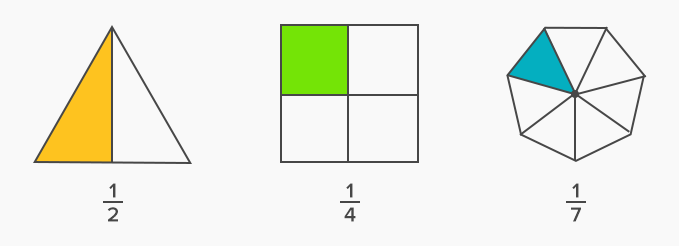Each unit fraction is a part of a whole or a part of 1. For example, 12 is a half of 1, 13 is a third of 1, 14 is a fourth of 1, and so on.

The easiest way is to break the larger fraction into a number of unit fractions.

For example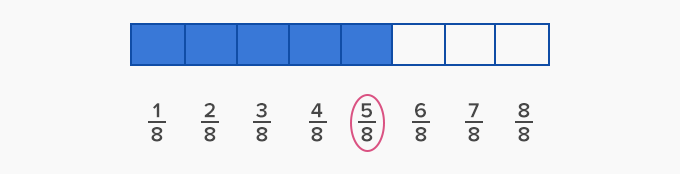We can see that 58 is the same as the five times of unit fraction 18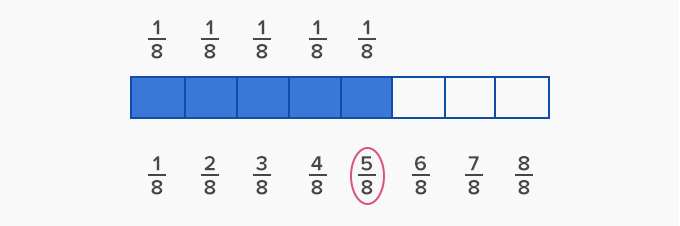18 + 18 + 18 + 18 + 18 = 58

Let’s take another example, consider the fraction 56, which means that it is 5 parts of a total of 6.

We can split this fraction into 5 parts each representing 1 part of a 6, that is 16 .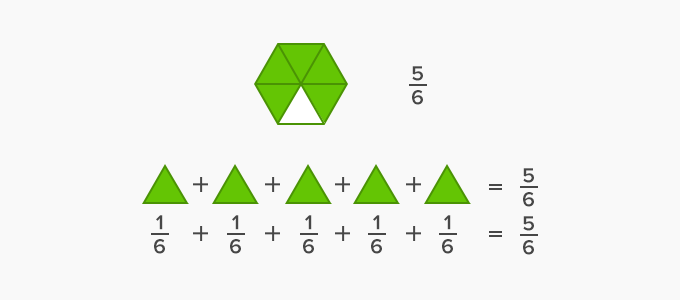Thus, to decompose a fraction, we have to break it up to equal the sum of the fraction 5.

B. Using the sum of the smaller fractions which are not all unit fractions

We can also decompose a fraction by using sum of smaller fractions.

56 can also be split up onto 16 , 16 and 36 or 26  and 36 or 16 and 46

Thus,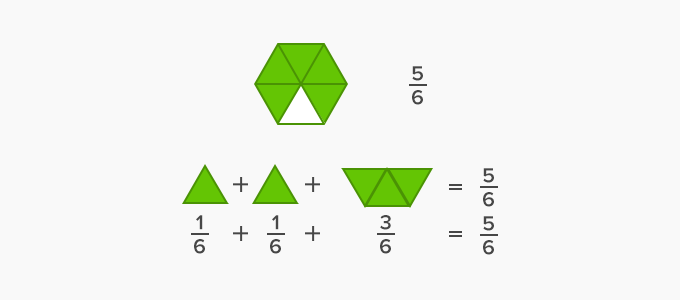Also,

56 = 26 + 36 = 13 + 12

Here we have simplified the fraction 26 = 13 and 312

## Decomposing mixed fractions

A mixed fraction is a whole number, and a proper fraction represented together. It represents a number between any two whole numbers.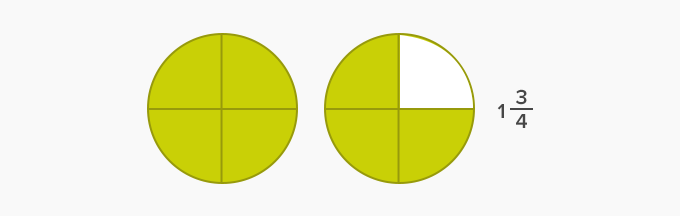The numerator and denominator are part of the proper fraction of which makes the mixed number.Let us split a mixed fraction.

13 = 2 + 13

A mixed fraction on splitting gives a whole number and a proper fraction.

Won Numerous Awards & Honors